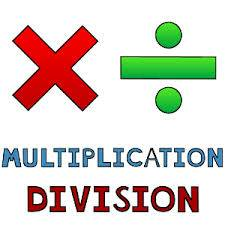# Tom works 14 hours a week for 10 weeks and earns a total of \$1,351.00. How much money does he make per hour?

Around \$9.65.

The math is pretty simple. 14 hours a week for 10 weeks is 140 hours, 1351 divided by 140 hours is 9.65.

Hope this helps!

1 Person thanked the writer.

This is the sort of calculation which shows the value of division and multiplication in everyday situations. Once you have the basic numbers in place, it's easy to work out the numbers you need, and we all need to know how much we earn per hour!To find out how much money Tom earns per hour, we first need to work out how many hours Tom has worked in total. For this, we use multiplication:

14 hours per week x 10 weeks in total =

14 x 10 = 140 hours

Next, we use division to calculate how much Tom earned during each of those 140 hours:

\$1351.00 earned in total divided by 140 hours =

1351 divided by 140 = 9.65

Therefore, Tom earned \$9.65 per hour over the ten weeks during which he earned a total of \$1351.00.

If you need more practice at multiplication and division, there are plenty of free worksheets available on line. Type in 'multiplication and division' into Google Images for a basic selection.

If you'd like to brush up your skills on sums, watch this YouTube tutorial:

thanked the writer.